# RS Aggarwal Solutions for Class 8 Maths Chapter 20 - Volume and Surface Area of Solids Exercise 20A

Students can get the pdf of RS Aggarwal Solutions for the Exercise 20A of Class 8 Maths Chapter 20, Volume and Surface Area of Solids from the link given below. Practising as many times as possible helps in building time management skills and also boosts the confidence level to achieve high marks. To accomplish this, BYJU’S expert has solved the question in different ways. This exercise deals with Volume and Surface area of cube and cuboids. By practising the RS Aggarwal Solutions for Class 8, students will be able to grasp the concepts entirely. Hence, students whose aim is to score high in Maths examinations are advised to go through RS Aggarwal Solutions for Class 8.

## Download PDF of RS Aggarwal Solutions for Class 8 Maths Chapter 20 – Volume And Surface Area Of Solids – Exercise 20A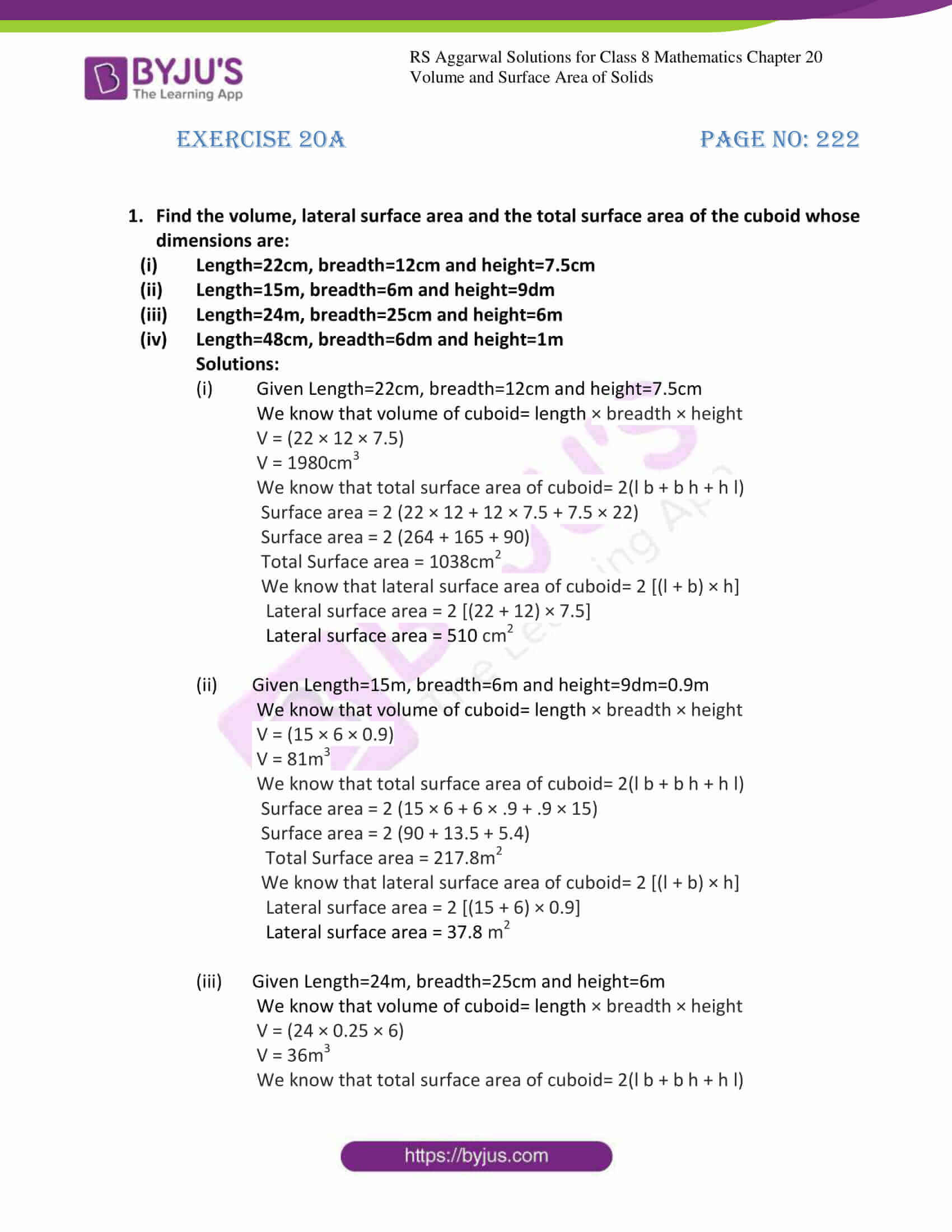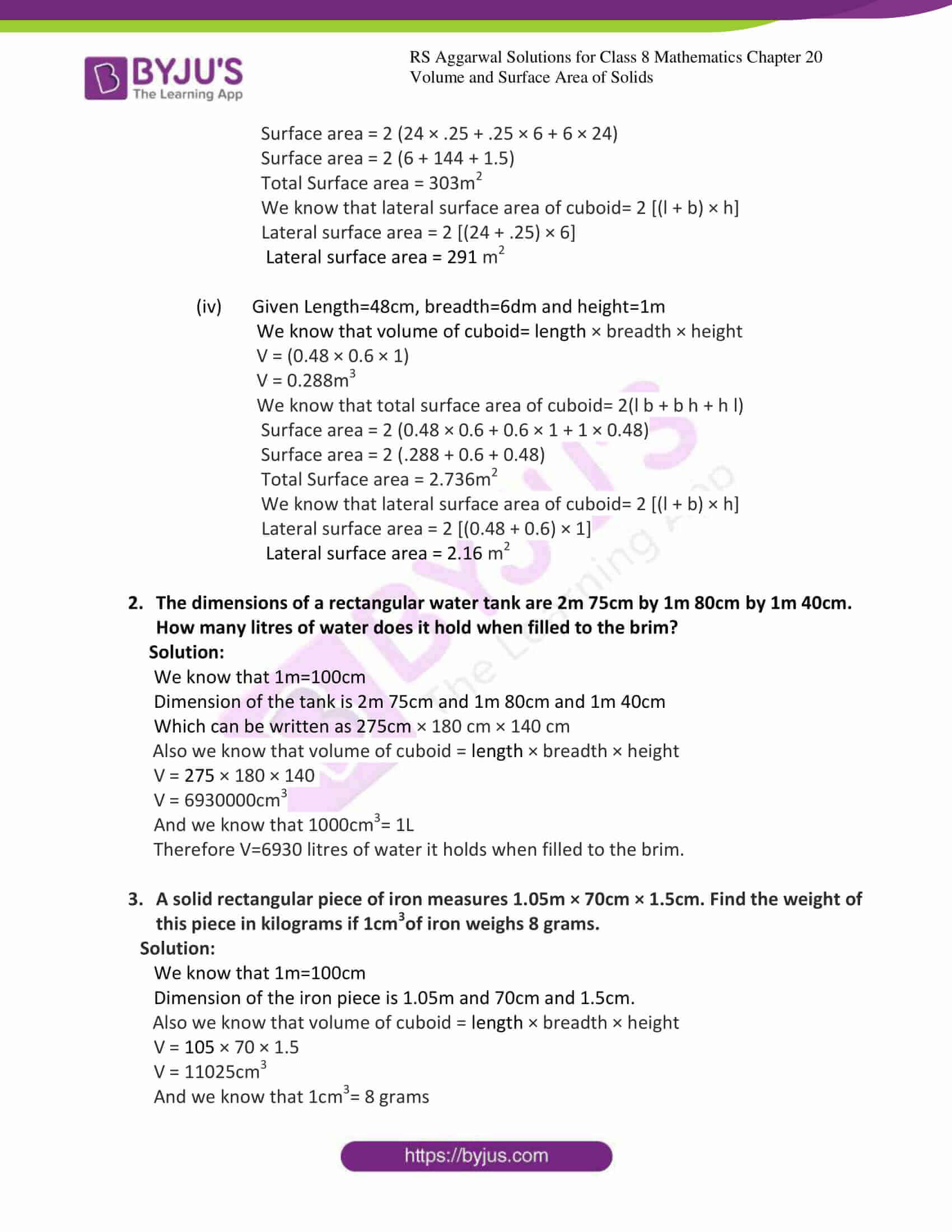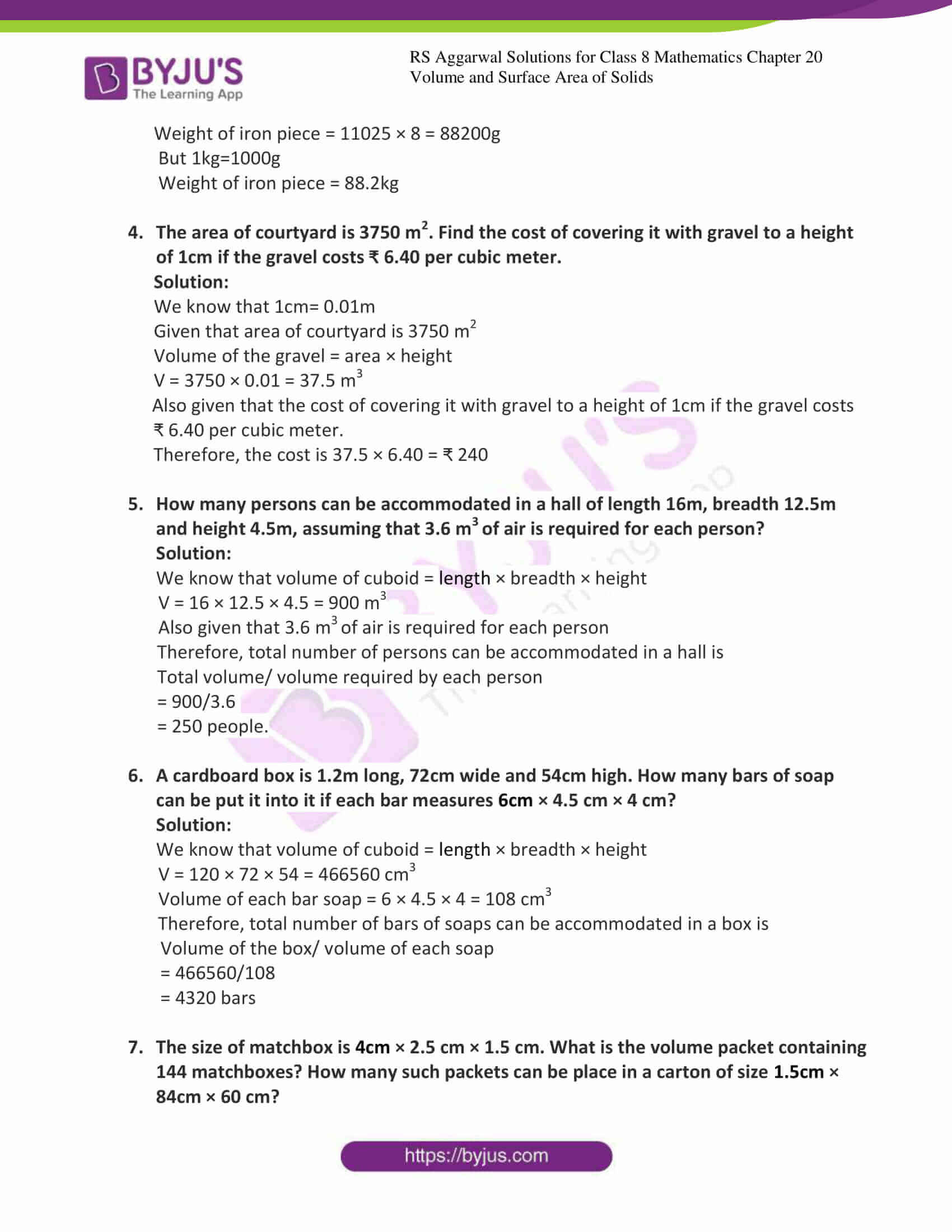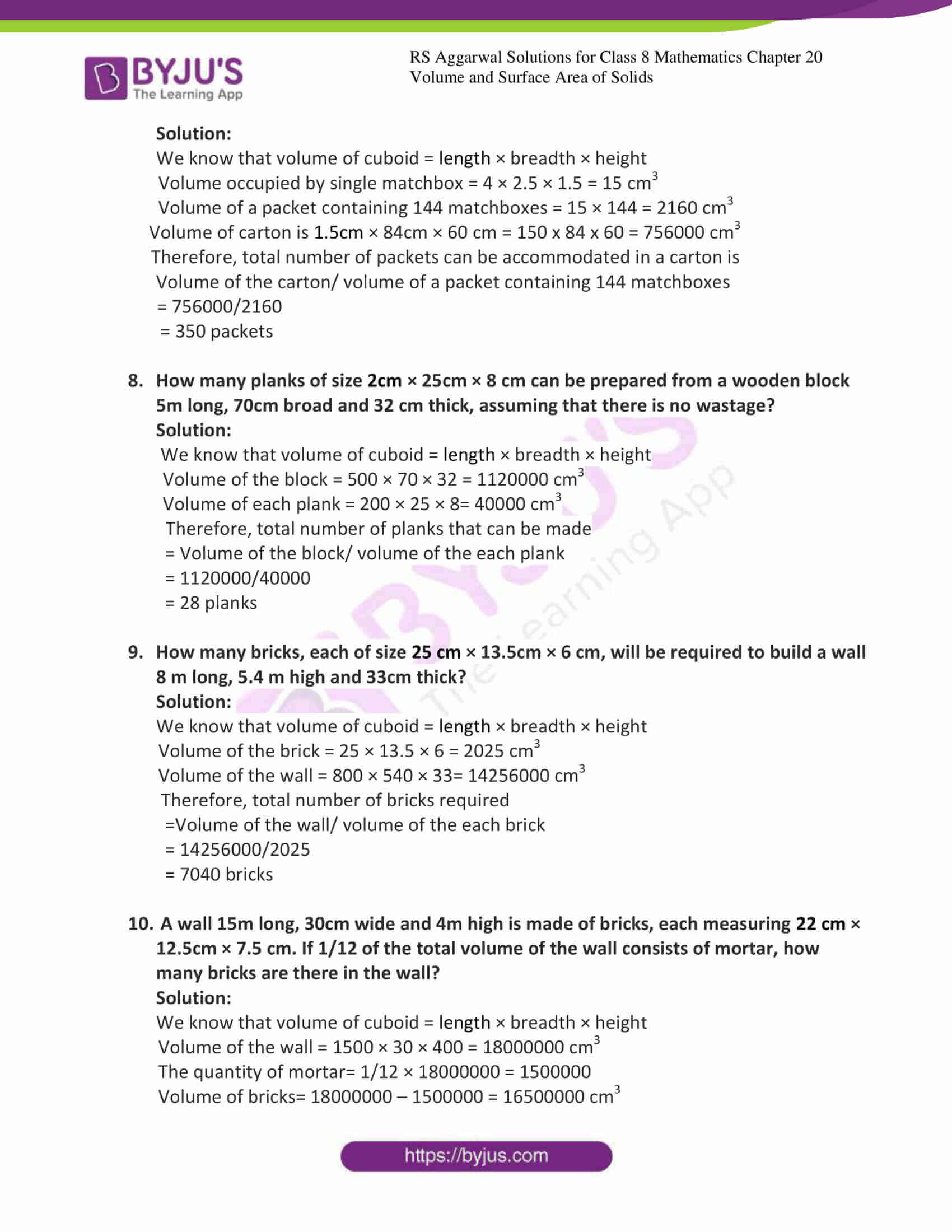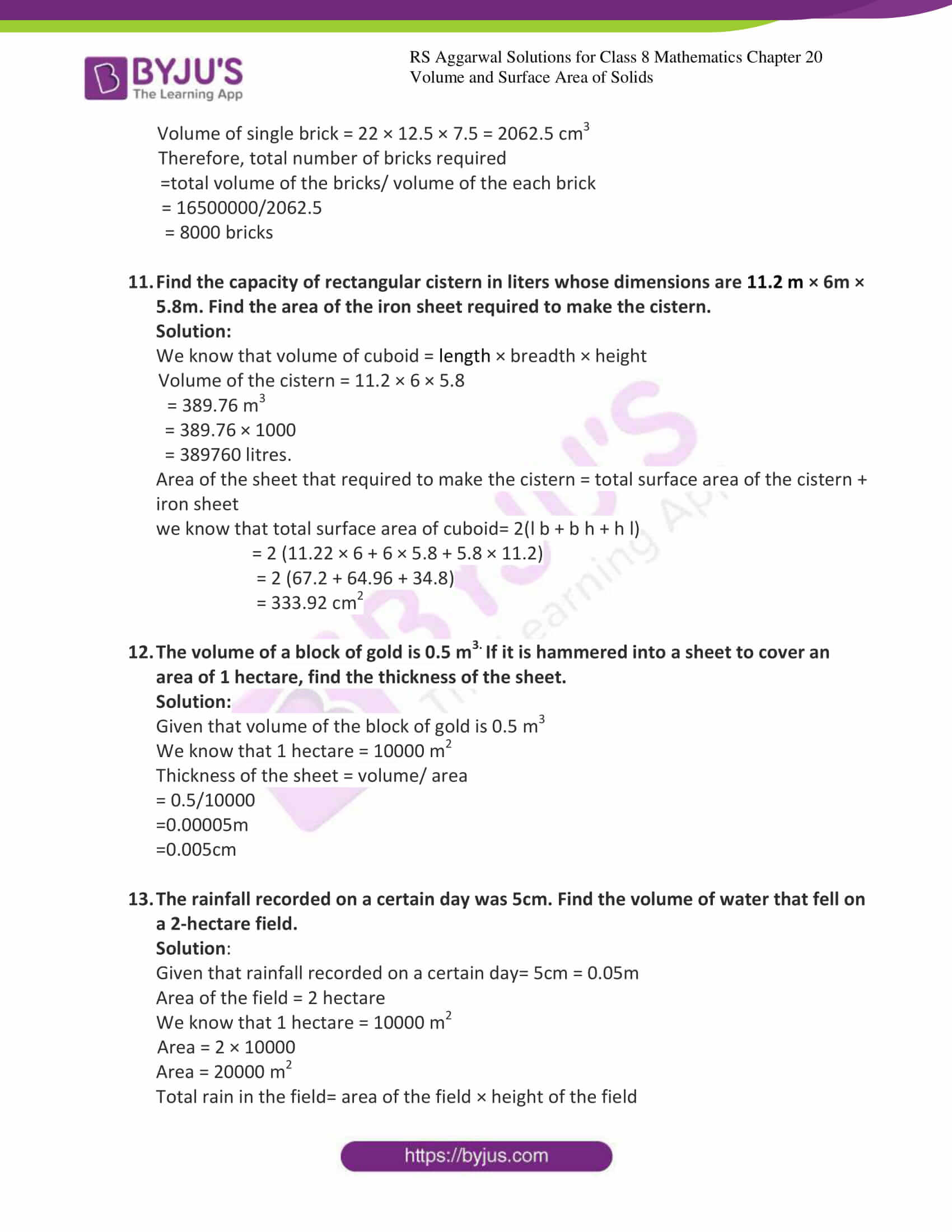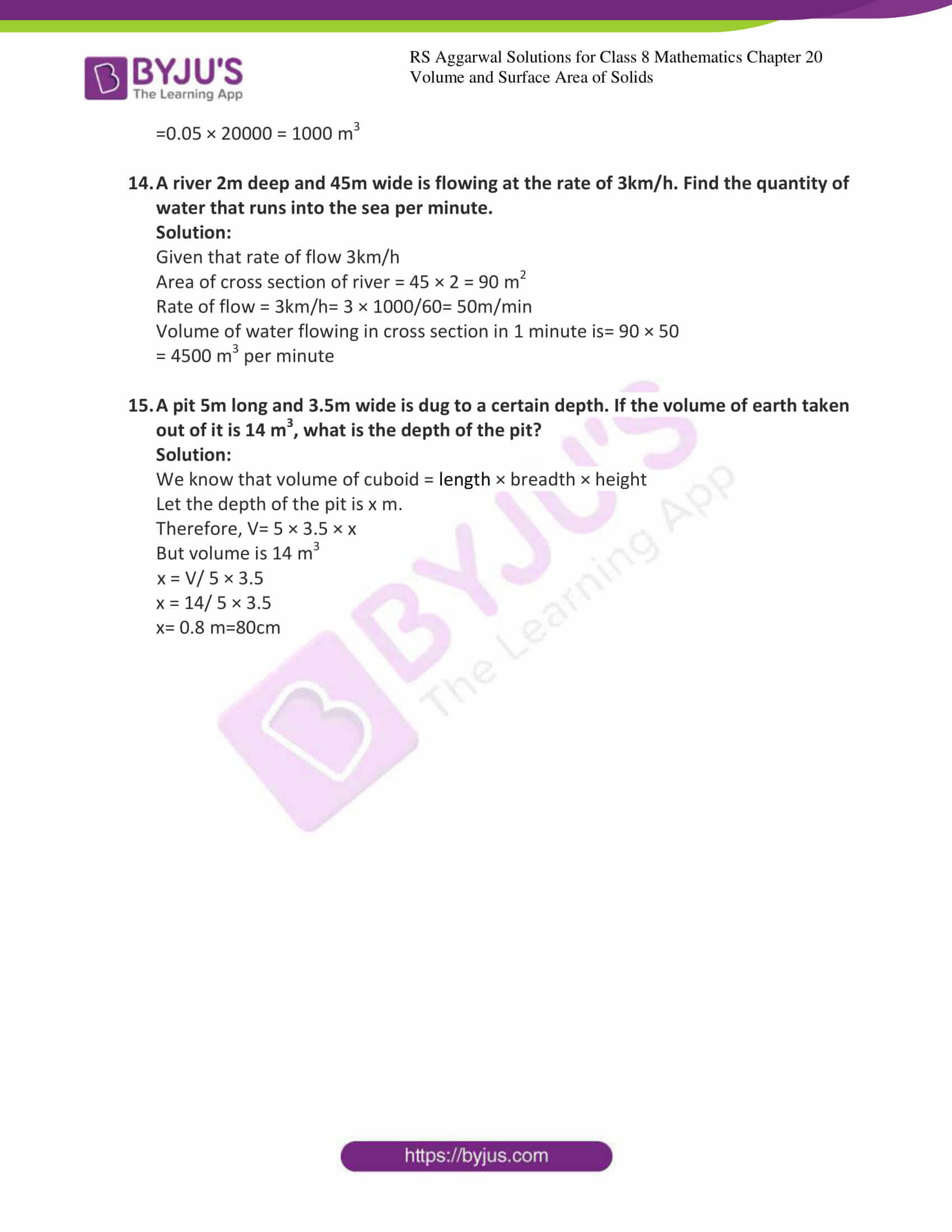### Access answers to Maths RS Aggarwal Solutions for Class 8 Chapter 20 – Volume and Surface Area of Solids Exercise 20A

Exercise 20A Page No: 222

1. Find the volume, lateral surface area and the total surface area of the cuboid whose dimensions are:

Solutions:

(i) Given Length=22cm, breadth=12cm and height=7.5cm

We know that volume of cuboid= length × breadth × height

V = (22 × 12 × 7.5)

V = 1980cm3

We know that total surface area of cuboid= 2(l b + b h + h l)

Surface area = 2 (22 × 12 + 12 × 7.5 + 7.5 × 22)

Surface area = 2 (264 + 165 + 90)

Total Surface area = 1038cm2

We know that lateral surface area of cuboid= 2 [(l + b) × h]

Lateral surface area = 2 [(22 + 12) × 7.5]

Lateral surface area = 510 cm2

(ii) Given Length=15m, breadth=6m and height=9dm=0.9m

We know that volume of cuboid= length × breadth × height

V = (15 × 6 × 0.9)

V = 81m3

We know that total surface area of cuboid= 2(l b + b h + h l)

Surface area = 2 (15 × 6 + 6 × .9 + .9 × 15)

Surface area = 2 (90 + 13.5 + 5.4)

Total Surface area = 217.8m2

We know that lateral surface area of cuboid= 2 [(l + b) × h]

Lateral surface area = 2 [(15 + 6) × 0.9]

Lateral surface area = 37.8 m2

(iii) Given Length=24m, breadth=25cm and height=6m

We know that volume of cuboid= length × breadth × height

V = (24 × 0.25 × 6)

V = 36m3

We know that total surface area of cuboid= 2(l b + b h + h l)

Surface area = 2 (24 × .25 + .25 × 6 + 6 × 24)

Surface area = 2 (6 + 144 + 1.5)

Total Surface area = 303m2

We know that lateral surface area of cuboid= 2 [(l + b) × h]

Lateral surface area = 2 [(24 + .25) × 6]

Lateral surface area = 291 m2

(iv) Given Length=48cm, breadth=6dm and height=1m

We know that volume of cuboid= length × breadth × height

V = (0.48 × 0.6 × 1)

V = 0.288m3

We know that total surface area of cuboid= 2(l b + b h + h l)

Surface area = 2 (0.48 × 0.6 + 0.6 × 1 + 1 × 0.48)

Surface area = 2 (.288 + 0.6 + 0.48)

Total Surface area = 2.736m2

We know that lateral surface area of cuboid= 2 [(l + b) × h]

Lateral surface area = 2 [(0.48 + 0.6) × 1]

Lateral surface area = 2.16 m2

2. The dimensions of a rectangular water tank are 2m 75cm by 1m 80cm by 1m 40cm. How many litres of water does it hold when filled to the brim?

Solution:

We know that 1m=100cm

Dimension of the tank is 2m 75cm and 1m 80cm and 1m 40cm

Which can be written as 275cm × 180 cm × 140 cm

Also we know that volume of cuboid = length × breadth × height

V = 275 × 180 × 140

V = 6930000cm3

And we know that 1000cm3= 1L

Therefore V=6930 litres of water it holds when filled to the brim.

3. A solid rectangular piece of iron measures 1.05m × 70cm × 1.5cm. Find the weight of this piece in kilograms if 1cm3of iron weighs 8 grams.

Solution:

We know that 1m=100cm

Dimension of the iron piece is 1.05m and 70cm and 1.5cm.

Also we know that volume of cuboid = length × breadth × height

V = 105 × 70 × 1.5

V = 11025cm3

And we know that 1cm3= 8 grams

Weight of iron piece = 11025 × 8 = 88200g

But 1kg=1000g

Weight of iron piece = 88.2kg

4. The area of courtyard is 3750 m2. Find the cost of covering it with gravel to a height of 1cm if the gravel costs ₹ 6.40 per cubic meter.

Solution:

We know that 1cm= 0.01m

Given that area of courtyard is 3750 m2

Volume of the gravel = area × height

V = 3750 × 0.01 = 37.5 m3

Also given that the cost of covering it with gravel to a height of 1cm if the gravel costs

₹ 6.40 per cubic meter.

Therefore, the cost is 37.5 × 6.40 = ₹ 240

5. How many persons can be accommodated in a hall of length 16m, breadth 12.5m and height 4.5m, assuming that 3.6 m3 of air is required for each person?

Solution:

We know that volume of cuboid = length × breadth × height

V = 16 × 12.5 × 4.5 = 900 m3

Also given that 3.6 m3 of air is required for each person

Therefore, total number of persons can be accommodated in a hall is

Total volume/ volume required by each person

= 900/3.6

= 250 people.

6. A cardboard box is 1.2m long, 72cm wide and 54cm high. How many bars of soap can be put it into it if each bar measures 6cm × 4.5 cm × 4 cm?

Solution:

We know that volume of cuboid = length × breadth × height

V = 120 × 72 × 54 = 466560 cm3

Volume of each bar soap = 6 × 4.5 × 4 = 108 cm3

Therefore, total number of bars of soaps can be accommodated in a box is

Volume of the box/ volume of each soap

= 466560/108

= 4320 bars

7. The size of matchbox is 4cm × 2.5 cm × 1.5 cm. What is the volume packet containing 144 matchboxes? How many such packets can be place in a carton of size 1.5cm × 84cm × 60 cm?

Solution:

We know that volume of cuboid = length × breadth × height

Volume occupied by single matchbox = 4 × 2.5 × 1.5 = 15 cm3

Volume of a packet containing 144 matchboxes = 15 × 144 = 2160 cm3

Volume of carton is 1.5cm × 84cm × 60 cm = 150 x 84 x 60 = 756000 cm3

Therefore, total number of packets can be accommodated in a carton is

Volume of the carton/ volume of a packet containing 144 matchboxes

= 756000/2160

= 350 packets

8. How many planks of size 2cm × 25cm × 8 cm can be prepared from a wooden block 5m long, 70cm broad and 32 cm thick, assuming that there is no wastage?

Solution:

We know that volume of cuboid = length × breadth × height

Volume of the block = 500 × 70 × 32 = 1120000 cm3

Volume of each plank = 200 × 25 × 8= 40000 cm3

Therefore, total number of planks that can be made

= Volume of the block/ volume of the each plank

= 1120000/40000

= 28 planks

9. How many bricks, each of size 25 cm × 13.5cm × 6 cm, will be required to build a wall 8 m long, 5.4 m high and 33cm thick?

Solution:

We know that volume of cuboid = length × breadth × height

Volume of the brick = 25 × 13.5 × 6 = 2025 cm3

Volume of the wall = 800 × 540 × 33= 14256000 cm3

Therefore, total number of bricks required

=Volume of the wall/ volume of the each brick

= 14256000/2025

= 7040 bricks

10. A wall 15m long, 30cm wide and 4m high is made of bricks, each measuring 22 cm × 12.5cm × 7.5 cm. If 1/12 of the total volume of the wall consists of mortar, how many bricks are there in the wall?

Solution:

We know that volume of cuboid = length × breadth × height

Volume of the wall = 1500 × 30 × 400 = 18000000 cm3

The quantity of mortar= 1/12 × 18000000 = 1500000

Volume of bricks= 18000000 – 1500000 = 16500000 cm3

Volume of single brick = 22 × 12.5 × 7.5 = 2062.5 cm3

Therefore, total number of bricks required

=total volume of the bricks/ volume of the each brick

= 16500000/2062.5

= 8000 bricks

11. Find the capacity of rectangular cistern in liters whose dimensions are 11.2 m × 6m × 5.8m. Find the area of the iron sheet required to make the cistern.

Solution:

We know that volume of cuboid = length × breadth × height

Volume of the cistern = 11.2 × 6 × 5.8

= 389.76 m3

= 389.76 × 1000

= 389760 litres.

Area of the sheet that required to make the cistern = total surface area of the cistern + iron sheet

we know that total surface area of cuboid= 2(l b + b h + h l)

= 2 (11.22 × 6 + 6 × 5.8 + 5.8 × 11.2)

= 2 (67.2 + 64.96 + 34.8)

= 333.92 cm2

12. The volume of a block of gold is 0.5 m3. If it is hammered into a sheet to cover an area of 1 hectare, find the thickness of the sheet.

Solution:

Given that volume of the block of gold is 0.5 m3

We know that 1 hectare = 10000 m2

Thickness of the sheet = volume/ area

= 0.5/10000

=0.00005m

=0.005cm

13. The rainfall recorded on a certain day was 5cm. Find the volume of water that fell on a 2-hectare field.

Solution:

Given that rainfall recorded on a certain day= 5cm = 0.05m

Area of the field = 2 hectare

We know that 1 hectare = 10000 m2

Area = 2 × 10000

Area = 20000 m2

Total rain in the field= area of the field × height of the field

=0.05 × 20000 = 1000 m3

14. A river 2m deep and 45m wide is flowing at the rate of 3km/h. Find the quantity of water that runs into the sea per minute.

Solution:

Given that rate of flow 3km/h

Area of cross section of river = 45 × 2 = 90 m2

Rate of flow = 3km/h= 3 × 1000/60= 50m/min

Volume of water flowing in cross section in 1 minute is= 90 × 50

= 4500 m3 per minute

15. A pit 5m long and 3.5m wide is dug to a certain depth. If the volume of earth taken out of it is 14 m3, what is the depth of the pit?

Solution:

We know that volume of cuboid = length × breadth × height

Let the depth of the pit is x m.

Therefore, V= 5 × 3.5 × x

But volume is 14 m3

x = V/ 5 × 3.5

x = 14/ 5 × 3.5

x= 0.8 m=80cm

### Access other exercises of RS Aggarwal Solutions for Class 8 Maths Chapter 20 – Volume and Surface Area of Solids

Exercise 20B Solutions 21 questions

Exercise 20C Solutions 30 questions

## RS Aggarwal Solutions for Class 8 Maths Chapter 20 – Volume and Surface Area of Solids Exercise 20A

Exercise 20A of RS Aggarwal Solutions for Class 8 Maths Chapter 20 Volume and Surface area of Solids deals with the basic concepts related to the total Surface Area of Solids. We can say that this exercise mainly deals with the definitions of solids that the students have learned in this Chapter. Some of the topics focused prior to exercise 20A include the following.

• Definition of cube
• Definition of cuboid
• The Volume of a Solid
• A standard unit of Volume

RS Aggarwal Solutions can help the students in exercising and learning each and every concept as it provides solutions to all questions asked in the RS Aggarwal textbook.